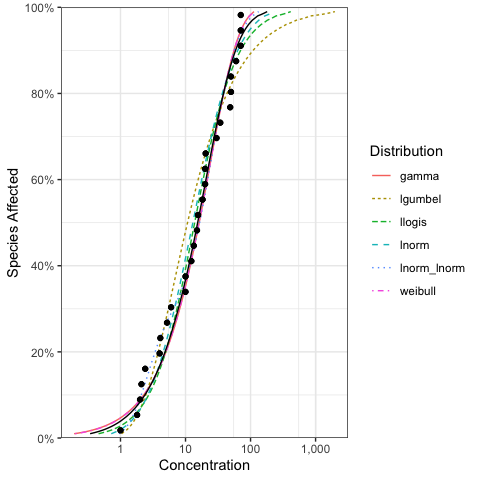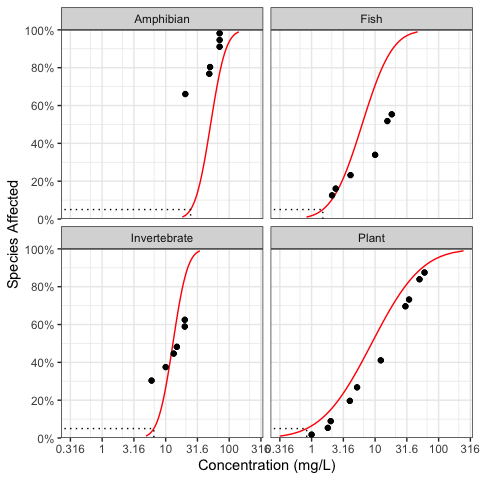## How can I plot the model averaged fit with individual fits?

library(ssddata)
library(ssdtools)
library(ggplot2)

dist <- ssdtools::ssd_fit_dists(ssddata::ccme_boron)
pred <- predict(dist, ci = FALSE)

ssdtools::ssd_plot_cdf(dist) +
geom_line(data = pred, aes(x = est, y = percent/100))## How do I fit distributions to multiple groups such taxa and/or chemicals?

An elegant approach using some tidyverse packages is demonstrated below.

library(ssddata)
library(ssdtools)
library(ggplot2)
library(dplyr)
library(tidyr)
library(purrr)

boron_preds <- nest(ccme_boron, data = c(Chemical, Species, Conc, Units)) %>%
mutate(
Fit = map(data, ssd_fit_dists, dists = "lnorm"),
Prediction = map(Fit, predict)
) %>%
unnest(Prediction)

The resultant data and predictions can then be plotted as follows.

ssd_plot(ccme_boron, boron_preds, xlab = "Concentration (mg/L)", ci = FALSE) +
facet_wrap(~Group)